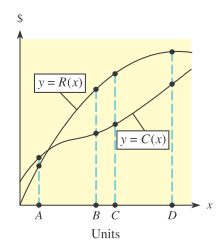# Marginal revenue and marginal cost The figure shows the graph of revenue function y = R ( x ) and cost function y = C ( x ) . At which of the four x-values shown is the profit largest? At which of the four x- values shown is the slope of the tangent to the revenue curve equal to the slope of the tangent to the cost curve? What is the relationship between marginal cost and marginal revenue when profit is at its maximum value?### Mathematical Applications for the ...

11th Edition
Ronald J. Harshbarger + 1 other
Publisher: Cengage Learning
ISBN: 9781305108042

#### Solutions

Chapter
Section### Mathematical Applications for the ...

11th Edition
Ronald J. Harshbarger + 1 other
Publisher: Cengage Learning
ISBN: 9781305108042
Chapter 10.3, Problem 32E
Textbook Problem
1 views

## Marginal revenue and marginal cost The figure shows the graph of revenue function y = R ( x ) and cost function y = C ( x ) . At which of the four x-values shown is the profit largest? At which of the four x-values shown is the slope of the tangent to the revenue curve equal to the slope of the tangent to the cost curve? What is the relationship between marginal cost and marginal revenue when profit is at its maximum value?This textbook solution is under construction.

### Still sussing out bartleby?

Check out a sample textbook solution.

See a sample solution

#### The Solution to Your Study Problems

Bartleby provides explanations to thousands of textbook problems written by our experts, many with advanced degrees!

Get Started